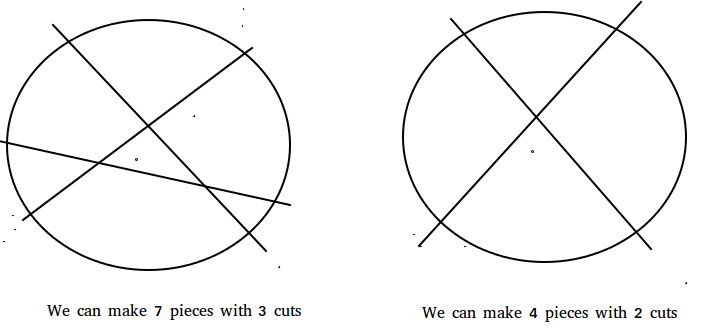# The Lazy Caterer’s Problem

Given an integer n, denoting the number of cuts that can be made on a pancake, find the maximum number of pieces that can be formed by making n cuts.
Examples :

```Input :  n = 1
Output : 2
With 1 cut we can divide the pancake in 2 pieces

Input :  2
Output : 4
With 2 cuts we can divide the pancake in 4 pieces

Input : 3
Output : 7
We can divide the pancake in 7 parts with 3 cuts

Input : 50
Output : 1276
```Recommended Practice

```Let f(n) denote the maximum number of pieces
that can be obtained by making n cuts.
Trivially,
f(0) = 1
As there'd be only 1 piece without any cut.

Similarly,
f(1) = 2

Proceeding in similar fashion we can deduce
the recursive nature of the function.
The function can be represented recursively as :
f(n) = n + f(n-1)

Hence a simple solution based on the above
formula can run in O(n). ```

We can optimize above formula.

```We now know ,
f(n) = n + f(n-1)

Expanding f(n-1) and so on we have ,
f(n) = n + n-1 + n-2 + ...... + 1 + f(0)

which gives,
f(n) = (n*(n+1))/2 + 1```

Hence with this optimization, we can answer all the queries in O(1).
Below is the implementation of above idea :

## C++

 `// A C++ program to find the solution to` `// The Lazy Caterer's Problem` `#include ` `using` `namespace` `std;`   `// This function receives an integer n` `// and returns the maximum number of` `// pieces that can be made form pancake` `// using n cuts` `int` `findPieces(``int` `n)` `{` `    ``// Use the formula` `    ``return` `(n * ( n + 1)) / 2 + 1;` `}`   `// Driver Code` `int` `main()` `{` `    ``cout << findPieces(1) << endl;` `    ``cout << findPieces(2) << endl;` `    ``cout << findPieces(3) << endl;` `    ``cout << findPieces(50) << endl;` `    ``return` `0;` `}`

## Java

 `// Java program to find the solution to` `// The Lazy Caterer's Problem` `import` `java.io.*;`   `class` `GFG ` `{` `    ``// This function returns the maximum ` `    ``// number of pieces that can be made` `    ``//  form pancake using n cuts` `    ``static` `int` `findPieces(``int` `n)` `    ``{` `        ``// Use the formula` `        ``return` `(n * (n + ``1``)) / ``2` `+ ``1``;` `    ``}` `    `  `    ``// Driver program to test above function` `    ``public` `static` `void` `main (String[] args) ` `    ``{` `        ``System.out.println(findPieces(``1``));` `        ``System.out.println(findPieces(``2``));` `        ``System.out.println(findPieces(``3``));` `        ``System.out.println(findPieces(``50``));` `    ``}` `}`   `// This code is contributed by Pramod Kumar`

## Python3

 `# A Python 3 program to ` `# find the solution to` `# The Lazy Caterer's Problem`   `# This function receives an ` `# integer n and returns the ` `# maximum number of pieces ` `# that can be made form ` `# pancake using n cuts` `def` `findPieces( n ):`   `    ``# Use the formula` `    ``return` `(n ``*` `( n ``+` `1``)) ``/``/` `2` `+` `1`   `# Driver Code` `print``(findPieces(``1``))` `print``(findPieces(``2``))` `print``(findPieces(``3``))` `print``(findPieces(``50``))`   `# This code is contributed` `# by ihritik`

## C#

 `// C# program to find the solution ` `// to The Lazy Caterer's Problem` `using` `System;`   `class` `GFG ` `{` `    ``// This function returns the maximum ` `    ``// number of pieces that can be made` `    ``// form pancake using n cuts` `    ``static` `int` `findPieces(``int` `n)` `    ``{` `        ``// Use the formula` `        ``return` `(n * (n + 1)) / 2 + 1;` `    ``}` `    `  `    ``// Driver code` `    ``public` `static` `void` `Main () ` `    ``{` `        ``Console.WriteLine(findPieces(1));` `        ``Console.WriteLine(findPieces(2));` `        ``Console.WriteLine(findPieces(3));` `        ``Console.Write(findPieces(50));` `    ``}` `}`   `// This code is contributed by Nitin Mittal.`

## PHP

 ``

## Javascript

 ``

Output :

```2
4
7
1276```

References : oeis.org
If you like GeeksforGeeks and would like to contribute, you can also write an article using write.geeksforgeeks.org or mail your article to review-team@geeksforgeeks.org. See your article appearing on the GeeksforGeeks main page and help other Geeks.